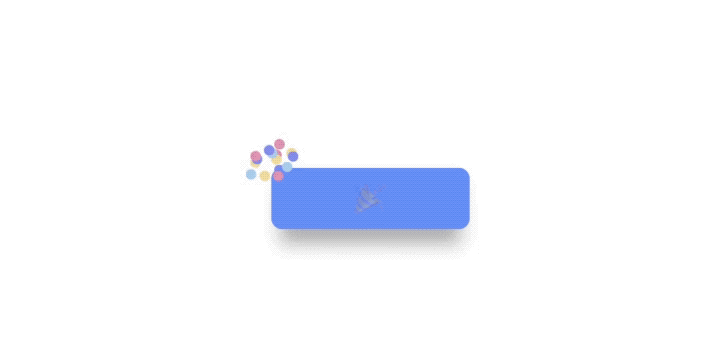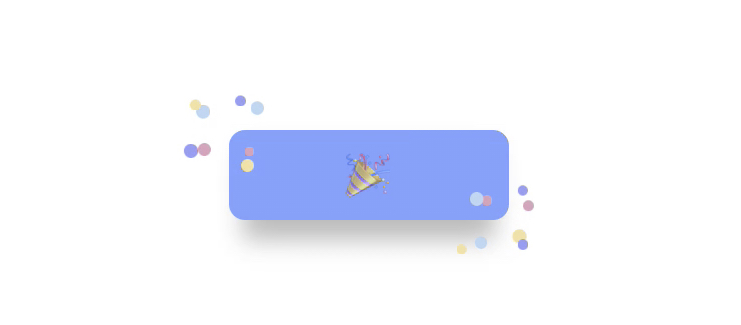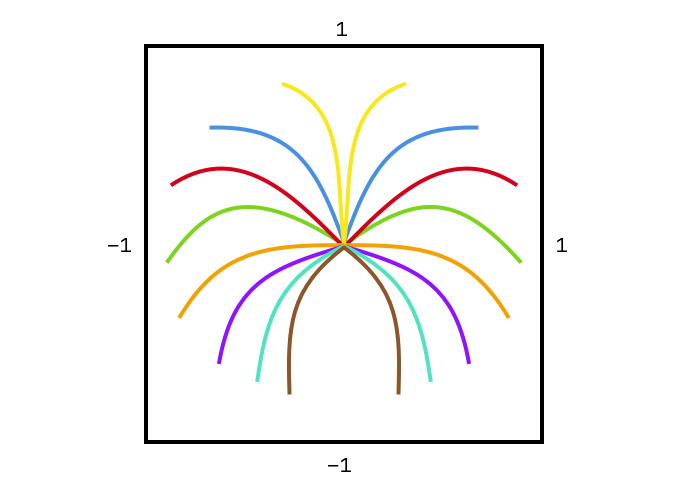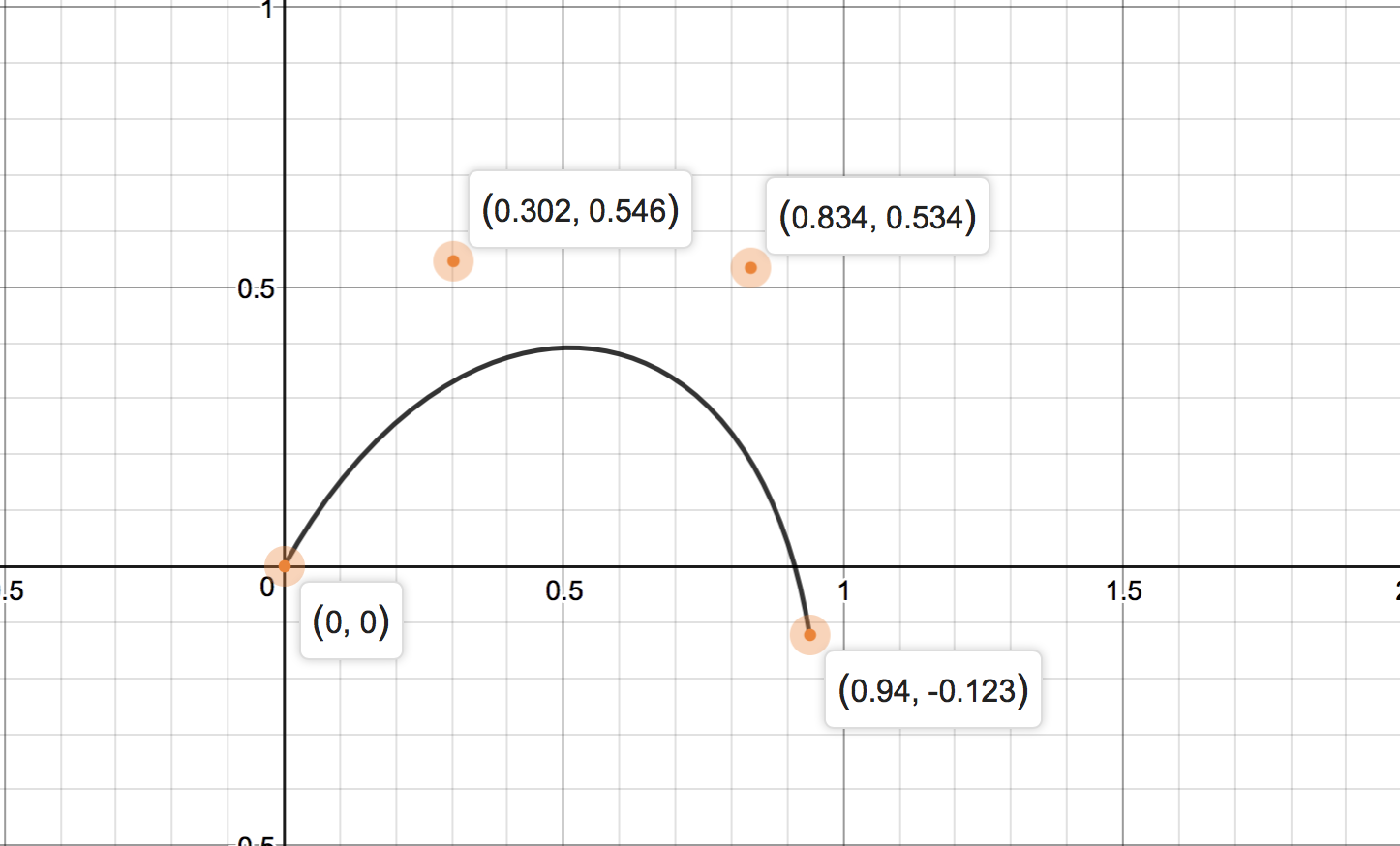# Add fireworks and sparks to a UIView

Do you like a little things that happens in apps you’re using? I looked at dribbble for inspirations and found a beautiful design of onboarding process where fireworks exploded (!) around important view when user changes settings or something. I wondered how difficult it is to implement, and some time later I finished it :)## Fireworks in details

Here is a detailed description of the effect. Fireworks should explode on a specific position around a view - presumably a button reacting on tap event. When it is tapped fireworks should explode close to the corners of the button and explosion should shift sparks from the explosion origin on their own trajectories.Love it! Makes my eyes happy and makes me want to tap the button all the time :) 🎉

Now let’s take a look at the animation. Behaviour of firework as a whole is similar for every generated firework. There are also small differencies in the trajectories and scale of sparks. Let’s break it down.

• There are two fireworks on each button tap,
• Each of them consists of 8 sparks,
• Each spark follow its own trajectory,
• Trajectories look similar but they’re not identical. Few goes to the right, few to the left, others to up and down looking from the explode origin standpoint.

### Sparks distribution

This firework has a simple spark distribution. Two sparks on each “view quarter” around the explosion origin. Two sparks in the top right, bottom right, bottom left and top left quarters.### Spark trajectory

Spark has a trajectory it is moving on. There is 8 sparks in a single firework so there is 8 trajectories needed at least. Ideally there should be more trajectories so we can random, so consecutive fireworks don’t look exactly as previous ones.I’ve created 4 trajectories for each quarter to add some randomness - twice more than sparks. For simplicity of calculations I normalized position of points creating trajectories. Presented trajectories differs a bit from what I finished with because I’ve used different tool to visualize implemented trajectories - but you can get the idea :)

## Implementation

Enough theory. Let’s put all the pieces together.

``````protocol SparkTrajectory {

/// Stores all points that defines a trajectory.
var points: [CGPoint] { get set }

/// A path representing trajectory.
var path: UIBezierPath { get }
}``````

Here is a protocol representing spark trajectory. Sharing common interface will make it easier to create various types of trajectories. I’ve decided to go with trajectories based on Cubic Bézier curve and added an init method so I can make it one line call. Path of this type must consists of four points. First and last points specifies start and finish position and two middle points are points to control a curvature of a path. You can use [demos] online math tool to play with Bézier curve.

``````/// Bezier path with two control points.
struct CubicBezierTrajectory: SparkTrajectory {

var points = [CGPoint]()

init(_ x0: CGFloat, _ y0: CGFloat,
_ x1: CGFloat, _ y1: CGFloat,
_ x2: CGFloat, _ y2: CGFloat,
_ x3: CGFloat, _ y3: CGFloat) {
self.points.append(CGPoint(x: x0, y: y0))
self.points.append(CGPoint(x: x1, y: y1))
self.points.append(CGPoint(x: x2, y: y2))
self.points.append(CGPoint(x: x3, y: y3))
}

var path: UIBezierPath {
guard self.points.count == 4 else { fatalError("4 points required") }

let path = UIBezierPath()
path.move(to: self.points)
path.addCurve(to: self.points, controlPoint1: self.points, controlPoint2: self.points)
return path
}
}``````Moving on. Next thing to implement is a factory that can random a trajectory. On the drawing above you can see trajectories grouped by color. I’ve only created top right and bottom right trajectories and mirror them. This is fine for a firework we’re about to launch 🚀

``````protocol SparkTrajectoryFactory {}

protocol ClassicSparkTrajectoryFactoryProtocol: SparkTrajectoryFactory {

func randomTopRight() -> SparkTrajectory
func randomBottomRight() -> SparkTrajectory
}

final class ClassicSparkTrajectoryFactory: ClassicSparkTrajectoryFactoryProtocol {

private lazy var topRight: [SparkTrajectory] = {
return [
CubicBezierTrajectory(0.00, 0.00, 0.31, -0.46, 0.74, -0.29, 0.99, 0.12),
CubicBezierTrajectory(0.00, 0.00, 0.31, -0.46, 0.62, -0.49, 0.88, -0.19),
CubicBezierTrajectory(0.00, 0.00, 0.10, -0.54, 0.44, -0.53, 0.66, -0.30),
CubicBezierTrajectory(0.00, 0.00, 0.19, -0.46, 0.41, -0.53, 0.65, -0.45),
]
}()

private lazy var bottomRight: [SparkTrajectory] = {
return [
CubicBezierTrajectory(0.00, 0.00, 0.42, -0.01, 0.68, 0.11, 0.87, 0.44),
CubicBezierTrajectory(0.00, 0.00, 0.35, 0.00, 0.55, 0.12, 0.62, 0.45),
CubicBezierTrajectory(0.00, 0.00, 0.21, 0.05, 0.31, 0.19, 0.32, 0.45),
CubicBezierTrajectory(0.00, 0.00, 0.18, 0.00, 0.31, 0.11, 0.35, 0.25),
]
}()

func randomTopRight() -> SparkTrajectory {
return self.topRight[Int(arc4random_uniform(UInt32(self.topRight.count)))]
}

func randomBottomRight() -> SparkTrajectory {
return self.bottomRight[Int(arc4random_uniform(UInt32(self.bottomRight.count)))]
}
}``````

I’ve named this firework a classic firework. Added a protocol for abstract spark trajectory factory, and a protocol for classic spark trajectory factory to be able to replace the classic factory with another factory.

As I mentioned before there are trajectories for two quarters I’ve created using desmos tool, and later on we’ll ask for a trajectory in a specific quarter top or bottom right.

Important notice: The y-axis* values are flipped, so if desmos tool presents positive value on Y-axis you should flip it to negative because of coordinates system in iOS - lower value closer the top of the screen.

Also it is worth to mention that for simplicity of later calculation each trajectory starts at (0, 0).

We have trajectories now. Let’s create a visual representation of a spark. Spark for classic firework will be a circle that has a color. Keeping the implementation more abstract will allow to create different spark views, e.g. with ducks images, or with pusheen cats in no time :)

``````class SparkView: UIView {}

final class CircleColorSparkView: SparkView {

init(color: UIColor, size: CGSize) {
super.init(frame: CGRect(origin: .zero, size: size))
self.backgroundColor = color
}

}
}

extension UIColor {

static var sparkColorSet1: [UIColor] = {
return [
UIColor(red:0.89, green:0.58, blue:0.70, alpha:1.00),
UIColor(red:0.96, green:0.87, blue:0.62, alpha:1.00),
UIColor(red:0.67, green:0.82, blue:0.94, alpha:1.00),
UIColor(red:0.54, green:0.56, blue:0.94, alpha:1.00),
]
}()
}``````

To create a spark view we need a factory and a data to fill a factory with. Basic data is a size of a spark and a index of a spark which defines what spark it is in a firework (for randomness).

``````protocol SparkViewFactoryData {

var size: CGSize { get }
var index: Int { get }
}

protocol SparkViewFactory {

func create(with data: SparkViewFactoryData) -> SparkView
}

class CircleColorSparkViewFactory: SparkViewFactory {

var colors: [UIColor] {
return UIColor.sparkColorSet1
}

func create(with data: SparkViewFactoryData) -> SparkView {
let color = self.colors[data.index % self.colors.count]
return CircleColorSparkView(color: color, size: data.size)
}
}``````

You can see that implementation of spark that look like pusheen cat should be easy. Let’s build a classic firework.

``````typealias FireworkSpark = (sparkView: SparkView, trajectory: SparkTrajectory)

protocol Firework {

/// Defines origin of firework.
var origin: CGPoint { get set }

/// Defines trajectory scale. Trajectory is normalized so it needs to be scaled up
/// before presenting on screen.
var scale: CGFloat { get set }

/// Defines size of a single spark.
var sparkSize: CGSize { get set }

/// Returns trajectories
var trajectoryFactory: SparkTrajectoryFactory { get }

/// Returns spark views
var sparkViewFactory: SparkViewFactory { get }

func sparkViewFactoryData(at index: Int) -> SparkViewFactoryData
func sparkView(at index: Int) -> SparkView
func trajectory(at index: Int) -> SparkTrajectory
}

extension Firework {

/// Helper method that return spark view and corresponding trajectory.
func spark(at index: Int) -> FireworkSpark {
return FireworkSpark(self.sparkView(at: index), self.trajectory(at: index))
}
}``````

Here is a abstract of a firework. To instantiate a firework what it needs:

• origin,
• scale,
• sparkSize
• trajectoryFactory
• sparkViewFactory

Before we go to classic implementation there is this concept of scaling trajectory that I didn’t mention before. As a spark trajectory is normalized and values of their points are close to <-1, 1> or similar, we want them to be proper size when spark is following the path. We have to scale the path up to cover bigger part of a screen. Also, we need to be able to flip a path horizontally so we can get trajectories for a left part of a classic spark, and be able to shift entire path a bit in specified direction (just for randomness and better control). Here are two methods that help us achieve the goal. I believe it’s self-explanatory.

``````extension SparkTrajectory {

/// Scales a trajectory so it fits to a UI requirements in terms of size of a trajectory.
/// Use it after all other transforms have been applied and before `shift`.
func scale(by value: CGFloat) -> SparkTrajectory {
var copy = self
(0..<self.points.count).forEach { copy.points[\$0].multiply(by: value) }
return copy
}

/// Flips trajectory horizontally
func flip() -> SparkTrajectory {
var copy = self
(0..<self.points.count).forEach { copy.points[\$0].x *= -1 }
return copy
}

/// Shifts a trajectory by (x, y). Applies to each point.
/// Use it after all other transformations have been applied and after `scale`.
func shift(to point: CGPoint) -> SparkTrajectory {
var copy = self
let vector = CGVector(dx: point.x, dy: point.y)
return copy
}
}``````

Okay, classic firework then.

``````class ClassicFirework: Firework {

/**
x     |     x
x  |   x
|
---------------
x |  x
x   |
|     x
**/

private struct FlipOptions: OptionSet {

let rawValue: Int

static let horizontally = FlipOptions(rawValue: 1 << 0)
static let vertically = FlipOptions(rawValue: 1 << 1)
}

private enum Quarter {

case topRight
case bottomRight
case bottomLeft
case topLeft
}

var origin: CGPoint
var scale: CGFloat
var sparkSize: CGSize

var maxChangeValue: Int {
return 10
}

var trajectoryFactory: SparkTrajectoryFactory {
return ClassicSparkTrajectoryFactory()
}

var classicTrajectoryFactory: ClassicSparkTrajectoryFactoryProtocol {
return self.trajectoryFactory as! ClassicSparkTrajectoryFactoryProtocol
}

var sparkViewFactory: SparkViewFactory {
return CircleColorSparkViewFactory()
}

private var quarters = [Quarter]()

init(origin: CGPoint, sparkSize: CGSize, scale: CGFloat) {
self.origin = origin
self.scale = scale
self.sparkSize = sparkSize
self.quarters = self.shuffledQuarters()
}

func sparkViewFactoryData(at index: Int) -> SparkViewFactoryData {
return DefaultSparkViewFactoryData(size: self.sparkSize, index: index)
}

func sparkView(at index: Int) -> SparkView {
return self.sparkViewFactory.create(with: self.sparkViewFactoryData(at: index))
}

func trajectory(at index: Int) -> SparkTrajectory {
let quarter = self.quarters[index]
let flipOptions = self.flipOptions(for: quarter)
let changeVector = self.randomChangeVector(flipOptions: flipOptions, maxValue: self.maxChangeValue)
return self.randomTrajectory(flipOptions: flipOptions).scale(by: self.scale).shift(to: sparkOrigin)
}

private func flipOptions(`for` quarter: Quarter) -> FlipOptions {
var flipOptions: FlipOptions = []
if quarter == .bottomLeft || quarter == .topLeft {
flipOptions.insert(.horizontally)
}

if quarter == .bottomLeft || quarter == .bottomRight {
flipOptions.insert(.vertically)
}

return flipOptions
}

private func shuffledQuarters() -> [Quarter] {
var quarters: [Quarter] = [
.topRight, .topRight,
.bottomRight, .bottomRight,
.bottomLeft, .bottomLeft,
.topLeft, .topLeft
]

var shuffled = [Quarter]()
for _ in 0..<quarters.count {
let idx = Int(arc4random_uniform(UInt32(quarters.count)))
shuffled.append(quarters[idx])
quarters.remove(at: idx)
}

return shuffled
}

private func randomTrajectory(flipOptions: FlipOptions) -> SparkTrajectory {
var trajectory: SparkTrajectory

if flipOptions.contains(.vertically) {
trajectory = self.classicTrajectoryFactory.randomBottomRight()
} else {
trajectory = self.classicTrajectoryFactory.randomTopRight()
}

return flipOptions.contains(.horizontally) ? trajectory.flip() : trajectory
}

private func randomChangeVector(flipOptions: FlipOptions, maxValue: Int) -> CGVector {
let values = (self.randomChange(maxValue), self.randomChange(maxValue))
let changeX = flipOptions.contains(.horizontally) ? -values.0 : values.0
let changeY = flipOptions.contains(.vertically) ? values.1 : -values.0
return CGVector(dx: changeX, dy: changeY)
}

private func randomChange(_ maxValue: Int) -> CGFloat {
return CGFloat(arc4random_uniform(UInt32(maxValue)))
}
}``````

Most of the code is implementation of a `Firework` protocol so it should be easy to understand. We pass correct factories here and there and using additional enums we random a trajectory for each spark.

There are few methods to add some randomness in terms of origin of a firework and origin of a single spark.

Also there is a concept of a quarter. There is an array of these and it is shuffled to make sure we do not always create sparks in the same quarters in the same quantity.

Okay, we’ve got the firework. How can we animate sparks then? Here comes a concept of spark animator.

``````protocol SparkViewAnimator {

func animate(spark: FireworkSpark, duration: TimeInterval)
}``````

What it does it takes a spark tuple which is a spark view and its trajectory as well as animation duration. Whatever happens in the method is up to us. My implementation is quite big but it just do three things: make the spark view follow the trajectory, scales it up and down (with some randomness), and finally changes it’s opacity. Simple. Also because of keeping this `SparkViewAnimator` abstract we can simply use different animators as we wish.

``````struct ClassicFireworkAnimator: SparkViewAnimator {

func animate(spark: FireworkSpark, duration: TimeInterval) {
spark.sparkView.isHidden = false // show previously hidden spark view

CATransaction.begin()

// Position
let positionAnim = CAKeyframeAnimation(keyPath: "position")
positionAnim.path = spark.trajectory.path.cgPath
positionAnim.calculationMode = kCAAnimationLinear
positionAnim.rotationMode = kCAAnimationRotateAuto
positionAnim.duration = duration

// Scale
let randomMaxScale = 1.0 + CGFloat(arc4random_uniform(7)) / 10.0
let randomMinScale = 0.5 + CGFloat(arc4random_uniform(3)) / 10.0

let fromTransform = CATransform3DIdentity
let byTransform = CATransform3DScale(fromTransform, randomMaxScale, randomMaxScale, randomMaxScale)
let toTransform = CATransform3DScale(CATransform3DIdentity, randomMinScale, randomMinScale, randomMinScale)
let transformAnim = CAKeyframeAnimation(keyPath: "transform")

transformAnim.values = [
NSValue(caTransform3D: fromTransform),
NSValue(caTransform3D: byTransform),
NSValue(caTransform3D: toTransform)
]

transformAnim.duration = duration
transformAnim.timingFunction = CAMediaTimingFunction(name: kCAMediaTimingFunctionEaseOut)
spark.sparkView.layer.transform = toTransform

// Opacity
let opacityAnim = CAKeyframeAnimation(keyPath: "opacity")
opacityAnim.values = [1.0, 0.0]
opacityAnim.keyTimes = [0.95, 0.98]
opacityAnim.duration = duration
spark.sparkView.layer.opacity = 0.0

// Group
let groupAnimation = CAAnimationGroup()
groupAnimation.animations = [positionAnim, transformAnim, opacityAnim]
groupAnimation.duration = duration

CATransaction.setCompletionBlock({
spark.sparkView.removeFromSuperview()
})

CATransaction.commit()
}
}``````

With the code presented we should be able to present firework on a specific view. I took it one step further and created a `ClassicFireworkController` that will manage all the work and let us make a single call to launch a firework.

This firework controller do one more thing. It can change a `zPosition` of a firework, so if we have a button it can launch some sparks behind and ahead of it for better look.

``````class ClassicFireworkController {

var sparkAnimator: SparkViewAnimator {
return ClassicFireworkAnimator()
}

func createFirework(at origin: CGPoint, sparkSize: CGSize, scale: CGFloat) -> Firework {
return ClassicFirework(origin: origin, sparkSize: sparkSize, scale: scale)
}

/// It allows fireworks to explodes in close range of corners of a source view
func addFireworks(count fireworksCount: Int = 1,
sparks sparksCount: Int,
around sourceView: UIView,
sparkSize: CGSize = CGSize(width: 7, height: 7),
scale: CGFloat = 45.0,
maxVectorChange: CGFloat = 15.0,
animationDuration: TimeInterval = 0.4,
canChangeZIndex: Bool = true) {
guard let superview = sourceView.superview else { fatalError() }

let origins = [
CGPoint(x: sourceView.frame.minX, y: sourceView.frame.minY),
CGPoint(x: sourceView.frame.maxX, y: sourceView.frame.minY),
CGPoint(x: sourceView.frame.minX, y: sourceView.frame.maxY),
CGPoint(x: sourceView.frame.maxX, y: sourceView.frame.maxY),
]

for _ in 0..<fireworksCount {
let idx = Int(arc4random_uniform(UInt32(origins.count)))
let origin = origins[idx].adding(vector: self.randomChangeVector(max: maxVectorChange))

let firework = self.createFirework(at: origin, sparkSize: sparkSize, scale: scale)

for sparkIndex in 0..<sparksCount {
let spark = firework.spark(at: sparkIndex)
spark.sparkView.isHidden = true

if canChangeZIndex {
let zIndexChange: CGFloat = arc4random_uniform(2) == 0 ? -1 : +1
spark.sparkView.layer.zPosition = sourceView.layer.zPosition + zIndexChange
} else {
spark.sparkView.layer.zPosition = sourceView.layer.zPosition
}

self.sparkAnimator.animate(spark: spark, duration: animationDuration)
}
}
}

private func randomChangeVector(max: CGFloat) -> CGVector {
return CGVector(dx: self.randomChange(max: max), dy: self.randomChange(max: max))
}

private func randomChange(max: CGFloat) -> CGFloat {
return CGFloat(arc4random_uniform(UInt32(max))) - (max / 2.0)
}
}``````

This controller does few things. It random a corner of a button to present a firework at. It adds some randomness to firework origin and create requested number of fireworks and sparks. Then it adds sparks to a source view, adjusts `zIndex` if possible and run the animator.

Almost all parameters have default value so you don’t need to care about them. You can call the controller just with this:

``self.fireworkController.addFireworks(count: 2, sparks: 8, around: button)``

And voilà!From this point it is easy to get a new type of firework that works like the following one. You just need to define new trajectories, create new firework and implement them to launch sparks as you wish. Putting all this code together in a controller simplifies launching fireworks wherever you want :) Or you can use a fountain firework I’ve included in tomkowz/fireworks on github.## Wrap up

Wasn’t easy but wasn’t difficult too. With proper analysis of the problem (or effect in that case) we can break it down to small pieces and put it together one piece at a time. Hopefully I’ll have a chance to use this effect in a future project 🎉

That’s it for today. Thanks for reading!

--